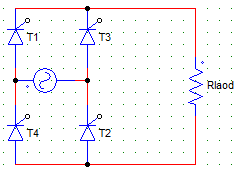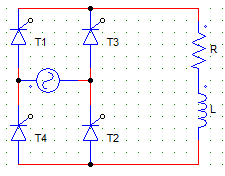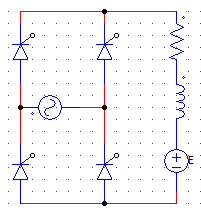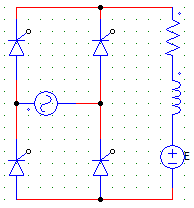# Test: Single Phase FW AC-DC - 2

## 20 Questions MCQ Test Power Electronics | Test: Single Phase FW AC-DC - 2

Description
Attempt Test: Single Phase FW AC-DC - 2 | 20 questions in 20 minutes | Mock test for Electrical Engineering (EE) preparation | Free important questions MCQ to study Power Electronics for Electrical Engineering (EE) Exam | Download free PDF with solutions
QUESTION: 1

### An SCR has the peak forward voltage = 1000 V. Find the maximum voltage that the SCR can handle if employed in a B-2 type full controlled converter circuit. Use factor of safety (FOS) = 2.5

Solution:

In M-2 type configuration maximum voltage handled is Vm
Therefore, 1000/2.5 = 400 V.

QUESTION: 2

### A single-phase full converter bridge, is connected to a RLE load. The source has a rms voltage of 230 V and the average load current is 10 A. Find the firing angle for which the power flows from AC source to the DC load. Consider E = 120 V, R = 0.4 Ω.

Solution:

We have,
Vo = E + IR
Vo = 2Vm/π x cosα
Substitute the given values to discover α = 53.208°.

QUESTION: 3

### A single-phase full converter bridge, is connected to a RLE load. The source has a rms voltage of 230 V and the average load current is 10 A. Find the firing angle for which the power flows from the DC load to the AC source. Consider E = 120 V, R = 0.4 Ω, L = 2 Henry.

Solution:

We have,
Vo = E + IR
Vo = 2Vm/π x cosα . . . (i)
Substitute the given values to discover α = 124°
Note that as the power is flowing from DC to AC E has to be negative.
E = -120V.
Use 1 to find the firing angle.

QUESTION: 4

For the below shown converter configuration, find the expression for the average value of voltage across the resister R with Vs = Vm sinωt and firing angle = α.Solution:

Vo = Vo = 1/π x [∫ Vm sinωt d(ωt)] where the integration would run from α to π.

QUESTION: 5

A single phase full controlled bridge converter is connected to load having R = 0.4 Ω, E = 120, L = 0.2 mH, Vs = 230V. The RMS value of load current is 10 A for a firing angle of 53.21°. Find the input power factor.

Solution:

Io = Irms = 10A
Vs x Irms x cosɸ = E Io + Irms2 R.

QUESTION: 6

A single phase full converter feeds power to a RLE load with R = 6 Ω and E = 60 V. Find the average value of the load current when the supply voltage is 230 V rms AC and a firing angle = 50°.

Solution:

Vo = 2Vm/π cosα = 133.084 V
I = Vo – E/R = 133.084-60 / 6 = 12.181 A.

QUESTION: 7

A single phase full wave mid-point SCR converter, uses a 230/200 V transformer with the centre tap on the secondary side. The P.I.V. per SCR is

Solution:

PIV for M-2 is Vm = √2Vs = √2 x 200.

QUESTION: 8

In a single phase full converter, for discontinuous load current and extinction angle β > π, each SCR conducts for

Solution:

Each device would conduct for β-α.

QUESTION: 9

A single-phase two pulse converter feeds RL load with a sufficient smoothing such that the load current does not fall to zero. If the resistance of the load circuit is increased then the

Solution:

If the resistance of the load circuit is increased then the ripple content of current increases.

QUESTION: 10

In case of controlled rectifiers, the nature of the load current (continues or discontinuous) depends upon the

Solution:

It depends on both as firing angle will decide how fast and how much current flows. The load R, Rl or RLE can also effect the current depending upon the values of L and E.

QUESTION: 11

In the circuit shown below, find the expression of average output voltage if due to some faults the SCR T3 gets permanently open circuited.Solution:

If one of the device gets O.C the circuit would behave just like a half-wave rectifier. Only Two SCRs T1 & T2 will operate, even T4 is useless in this case.
Vo = 1/2π x [ ∫ Vm sinωt d(ωt) ] where the integration would run from α to π+α.

QUESTION: 12

A single phase full converter, feeds power to a RLE load with R = 6 Ω and E = 60 V. The supply voltage is 230 V AC and the firing angle is 50°. Find the power delivered to the battery.

Solution:

Vo = 2Vm/π cosα = 133.084 V
I = Vo – E/R = 133.084-60 / 6 = 12.181 A
Power delivered = E x I = 60 x 12.181 = 730.86 Watts.

QUESTION: 13

In a single phase full converter with resistive load and firing angle α, the load current is

Solution:

The current starts to flow from α, π+α, …

QUESTION: 14

In a single phase semi converter with resistive load and a firing angle α, each SCR and freewheeling diode would conduct for

Solution:

FD does not come into the picture for resistive loads.

QUESTION: 15

A single phase full converter has average & peak voltage values of 133 V and 325 V respectively. Find the value of the firing angle.

Solution:

Vm = 325V
Vo = 2Vm/π cosα = 133 V.

QUESTION: 16

A freewheeling diode placed across a RL load provides

Solution:

FD improves efficiency by providing freewheeling action which increase the power delivered to the load

QUESTION: 17

A single phase full converter has discontinues load current. The converter is supplying a dc shunt motor (R-L-E) load. When the current falls to zero the output voltage is

Solution:

As the load is RLE output voltage will always exists even if the current goes to zero due to E.

QUESTION: 18

A single phase full converter has discontinues load current. The converter is supplying a DC shunt motor (R-L-E) load. When the current falls to zeroSolution:

The current falls to zero when the L has discharged completely and the devices are not yet fired.

QUESTION: 19

For the circuit shown below to act as a line commutated inverterSolution:

For inverter operation the firing angle should be above 180 and E should be reversed.

QUESTION: 20

If in the below given converter configuration, any 3 of the 4 SCRs are replaced by diodes

Solution:

If diodes are put in place of any 3 SCRs the conduction angle would increase and hence the voltage.Use Code STAYHOME200 and get INR 200 additional OFF Use Coupon Code